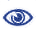# Prague Stringology Conference 2015

Computing Left-Right Maximal Generic Words

 Abstract: The maximal generic words problem was proposed by Kucherov et al. (SPIRE 2012). Let D be a set of documents. In this problem, given a pattern P and a threshold d ≤ |D|, we want to compute all right-maximal extensions of P which occur in at least d distinct documents. They proposed an O(n)-space data structure which can solve this problem in O(|P| + rocc) time where n is the total length of documents in D and rocc is the number of right-maximal extensions of P. The data structure can be constructed in O(n) time. In this paper, we propose a more generalized problem. Given a pattern P and a threshold d ≤ |D|, we want to compute all left-right-maximal extensions of P which occur in at least d distinct documents. We propose an O(n log n)-space data structure which can solve this problem in O(|P| + occ log2n + rocc log n) time where occ is the number of left-right-maximal extensions of P.

 Download paper:PostScript PDF BibTeX reference
 Download presentation: